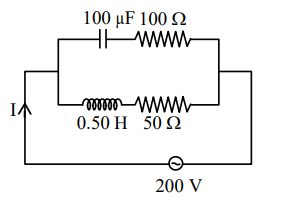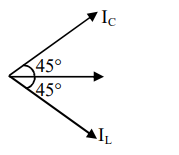Deepak Scored 45->99%ile with Bounce Back Crack Course. You can do it too!

# In the given circuit the

Question:

In the given circuit the $\mathrm{AC}$ source has $\omega=100 \mathrm{rad} \mathrm{} \mathrm{s}^{-1}$. Considering the inductor and capacitor to be ideal, what will be the current I flowing through the circuit?1. $5.9 \mathrm{~A}$

2. $4.24 \mathrm{~A}$

3. $0.94 \mathrm{~A}$

4. $6 \mathrm{~A}$

Correct Option: , 2

Solution:

$Z_{C}=\sqrt{\left(\frac{1}{\omega C}\right)^{2}+R^{2}}$

$=\sqrt{\left(\frac{1}{100 \times 100 \times 10^{-6}}\right)^{2}+100^{2}}$

$Z_{\mathrm{C}}=\sqrt{(100)^{2}+(100)^{2}}$

$=100 \sqrt{2}$

$Z_{L}=\sqrt{(\omega L)^{2}+R^{2}}$

$\sqrt{(100 \times 0.5)^{2}+50^{2}}$

$=50 \sqrt{2}$

$i_{C}=\frac{200}{Z_{C}}=\frac{200}{100 \sqrt{2}}=\sqrt{2}$

$i_{L}=\frac{200}{Z_{L}}=\frac{200}{50 \sqrt{2}}=2 \sqrt{2}$

$\cos \phi_{1}=\frac{100}{10 \sqrt{2}}=\frac{1}{\sqrt{2}} \Rightarrow \phi_{1}=45^{\circ}$

$\cos \phi_{2}=\frac{50}{50 \sqrt{2}}=\frac{1}{\sqrt{2}} \Rightarrow \phi_{2}=45^{\circ}$$\mathrm{I}=\sqrt{\mathrm{I}_{\mathrm{C}}^{2}+\mathrm{I}_{\mathrm{L}}^{2}}$

$=\sqrt{2+8}$

$=\sqrt{10}$

$\mathrm{I}=3.16 \mathrm{~A}$

Ans. $3.16$